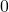# Theory

The trigonometric values for angles betweenanddegrees can be defined as dimensions on the unit circle as shown below. Sine and cosine are lengths of half chords, tangent is a measurement on the tangent line. Compare these values to those given on your calculator. (Click ‘show values’ twice).

With this diagram (without clicking on ‘show values’), you can order the trig ratios according to size (although for some angles this is more obvious than others). You can also make trig pairs – for example,. You can understand that sine and cosine have both a minimum value and a maximum value, however tangent does not have a maximum value. The tangent of 90 degrees is undefined.

The radius along with the green and the red half chords form a right angled triangle. We use these values to calculate lengths and angles on right angled triangles.

Note that for angles measuring from negative infinity to positive inifinity, it is better to define the trigonometric values as positions on the unit circle (or tangent line), as this allows for both positive and negative values.

# Solving Triangles

Unit 2 Review 1 kuta worksheet  with answers -sides and angles

Use this applet to practice simple triangle problems: